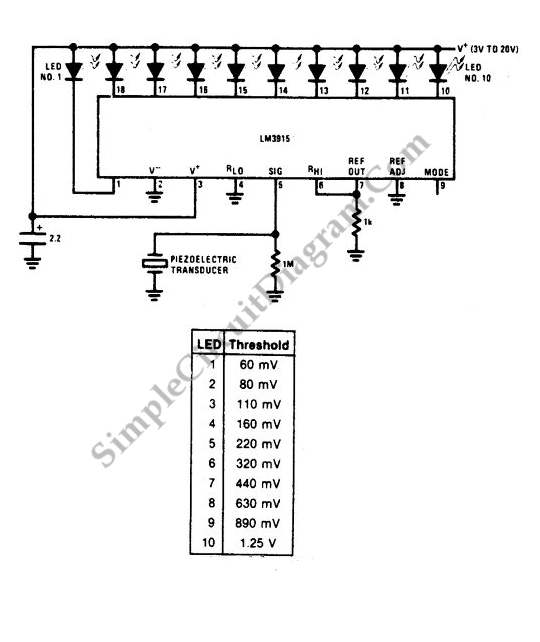# LM3915 Vibration Meter

A vibration meter circuit shown in the following schematic diagram uses LM3915 a the main active component. This vibration meter uses a piezoelectric transducer to pick up the vibration. The logarithmic characteristic of LM3915 make this meter circuit works for wide range measurement, suitable for measuring engine block vibration or other system where the dynamics is wide. Here is the schematic diagram of the circuit:[Circuit’s schematic diagram source: National Semiconductor Application Notes]